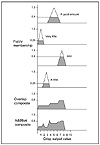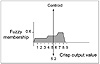# 133.C++ Neural Networks and Fuzzy Logic by Valluru B. Rao M&T Books, IDG Books Worldwide, Inc. ISBN: 1558515526   Pub Date: 06/01/95

Assume that at a particular point in time, LevelSense = 7.0 and TempSense = 65. These are the crisp inputs directly from the sensors. With fuzzification (refer to Chapter 3 for a review), assume that you get the following fuzzy memberships:

crisp input — LevelSense = 7.0
fuzzy outputs with membership values -
Medium: 0.4
Large: 0.6
all others : 0.0
crisp input — TempSense=65
fuzzy outputs with membership values -
Medium: 0.75
Large: 0.25
all others: 0.0

This results in four rules firing:

1.  TempSense = Medium (0.75) AND LevelSense = Medium (0.4)
2.  TempSense = Large (0.25) AND LevelSense = Medium (0.4)
3.  TempSense = Medium (0.75) AND LevelSense = Large (0.6)
4.  TempSense = Large (0.25) AND LevelSense = Large (0.6)

First you must determine, for each of the AND clauses in the IF–THEN rules, what the output should be. This is done by the conjunction or minimum operator. So for each of these rules you have the following firing strengths:

1.  (0.75) ⊥ (0.4) = 0.4
2.  (0.25) ⊥ (0.4) = 0.25
3.  (0.75) ⊥ (0.6) = 0.6
4.  (0.25) ⊥ (0.6) = 0.25

By using the fuzzy rule base and the strengths assigned previously, we find the rules recommend the following output values (with strengths) for HeatKnob:

1.  AGoodAmount (0.4)
2.  VeryLittle (0.25)
3.  ALot (0.6)
4.  ALittle (0.25)

Now we must combine the recommendations to arrive at a single crisp value. First, we will use the fuzzy Or method of defuzzification. Here we use a disjunction or maximum operator to combine the values. We obtain the following:

`      (0.4) ⊦ (0.25) ⊦ (0.6) ⊦ (0.25) = 0.6 `

The crisp output value for HeatKnob would then be this membership value multiplied by the range of the output variable, or (0.6) (10-0) = 6.0.

Another way of combining the outputs is with the centroid method. With the centroid method, there are two variations, the overlap composition method and the additive composition method. To review, we have the following output values and strengths.

1.  AGoodAmount (0.4)
2.  VeryLittle (0.25)
3.  ALot (0.6)
4.  ALittle (0.25)

We use the strength value and fill in the particular triangular membership function to that strength level. For example, for the first rule, we fill the triangular membership function, AGoodAmount to the 0.4 level. We then cut off the top of the triangle (above 0.4). Next we do the same for the other rules. Finally we align the truncated figures as shown in Figure 16.6 and combine them according to the overlap composition method or the additive composition method (Kosko). You can see the difference in these two composition methods in Figure 16.6. The overlap method simply superimposes all of the truncated triangles onto the same area. You can lose information with this method. The additive method adds the geometrical figures on top of each other.Figure 16.6  Defuzzification with the Centroid approach: Overlap and Additive composition.

In order to get a crisp value, you take the centroid or center of gravity, of the resulting geometrical figure. Let us do this for the overlap method figure. The centroid is a straight edge that can be placed through the figure to have it perfectly balanced; there is equal area of the figure on either side of the straight edge, as shown in Figure 16.7. Splitting up the geometry into pieces and summing all area contributions on either side of the centroid, we get a value of 5.2 for this example. This is already in terms of the crisp output value range:

`      HeatKnob = 5.2 `Figure 16.7  Finding the centroid.

This completes the design for the simple example we chose. We conclude with a list of the advantages and disadvantages of FLCs.

The following list adapted from McNeill and Thro shows the advantages and disadvantages to FLCs for control systems as compared to more traditional control systems.

Relates input to output in linguistic terms, easily understood
Allows for rapid prototyping because the system designer doesn’t need to know everything about the system before starting
Cheaper because they are easier to design
Increased robustness
Simplify knowledge acquisition and representation
A few rules encompass great complexity
Can achieve less overshoot and oscillation
Can achieve steady state in a shorter time interval

Hard to develop a model from a fuzzy system
Require more fine tuning and simulation before operational
Have a stigma associated with the word fuzzy (at least in the Western world); engineers and most other people are used to crispness and shy away from fuzzy control and fuzzy decision making

### Summary

Fuzzy logic applications are many and varied. You got an overview of the different applications areas that exist for fuzzy logic, from the control of washing machines to fuzzy logic based cost benefit analysis. Further you got details on two application domains: fuzzy databases and fuzzy control.

This chapter dealt with extending database models to accommodate fuzziness in data attribute values and in queries as well. You saw fuzzy relations; in particular, similarity and resemblance relations, and similarity classes were reviewed. You found how possibility distributions help define fuzzy databases. You also learned what fuzzy events are and how to calculate fuzzy means, fuzzy variances, and fuzzy conditional expectations. Concepts related to linear possibility regression model were presented.

The chapter presented the design of a simple fuzzy logic control (FLC) system to regulate the temperature of water in a hot water heater. The components of the FLC were discussed along with design procedures. The advantages of FLC design include rapid prototyping ability and the capability to solve very nonlinear control problems without knowing details of the nonlinearities.C++ Neural Networks and Fuzzy Logic
ISBN: 1558515526
EAN: 2147483647
Year: 1995
Pages: 139

Similar book on Amazon